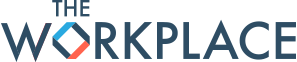## Users by Reputation Level

0

(click on this box to dismiss)Q&A for members of the workforce navigating the professional setting

``````SELECT
COUNT(*) AS "Active Users",
SUM(CASE WHEN Reputation >= 0 and Reputation < 150 THEN 1 ELSE 0 END) AS "0-150",
SUM(CASE WHEN Reputation >= 150 and Reputation < 500 THEN 1 ELSE 0 END) AS "150-500",
SUM(CASE WHEN Reputation >= 500 and Reputation < 1000 THEN 1 ELSE 0 END) AS "500-1k",
SUM(CASE WHEN Reputation >= 1000 and Reputation < 2000 THEN 1 ELSE 0 END) AS "1k-2k",
SUM(CASE WHEN Reputation >= 2000 and Reputation < 3000 THEN 1 ELSE 0 END) AS "2k-3k",
SUM(CASE WHEN Reputation >= 3000 and Reputation < 5000 THEN 1 ELSE 0 END) AS "3k-5k",
SUM(CASE WHEN Reputation >= 5000 and Reputation < 10000 THEN 1 ELSE 0 END) AS "5k-10k",
SUM(CASE WHEN Reputation >= 10000 and Reputation < 15000 THEN 1 ELSE 0 END) AS "10k-15k",
SUM(CASE WHEN Reputation >= 15000 and Reputation < 20000 THEN 1 ELSE 0 END) AS "15k-20k",
SUM(CASE WHEN Reputation >= 20000 THEN 1 ELSE 0 END) AS ">20k"
FROM Users
WHERE CreationDate <= '2014-04-08'``````

### Enter Parameters

Options:
-Hold tight while we fetch your results
:records returned in :time ms:cached